# Fractional integration and differentiation

(diff) ← Older revision | Latest revision (diff) | Newer revision → (diff)

An extension of the operations of integration and differentiation to the case of fractional powers. Letbe integrable on the interval, letbe the integral ofalong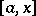, while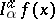is the integral of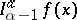along,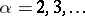. One then has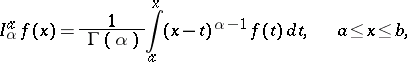(1)

whereis the gamma-function. The right-hand side makes sense for every. The relation (1) defines the fractional integral (or the Riemann–Liouville integral) of orderofwith starting point. The operator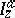was studied by B. Riemann (1847) for complex values of the parameter. The operator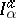is linear and has the semi-group property: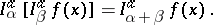The operation inverse to fractional integration is known as fractional differentiation: If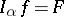, thenis the fractional derivative of orderof. If, Marchaut's formula applies:The concept of fractional integration and differentiation was first introduced by J. Liouville (1832); he studied, in particular, the operator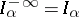,: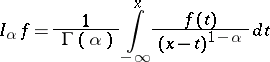(subject to appropriate restrictions on; cf. , which also contains estimates of the operator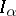in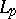).

The following definition (H. Weyl, 1917) is convenient for an integrable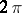-periodic functionwith zero average value over the period. Ifthen the Weyl integralof order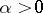ofis defined by the formula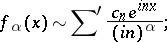(2)

and the derivative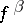of order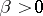is defined by the equation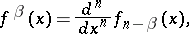whereis the smallest integer larger than(it should be noted that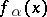coincides with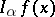).

These definitions were further developed in the framework of the theory of generalized functions. For periodic generalized functionsthe operation of fractional integration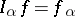is realized according to formula (2) for all real(ifis negative,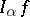coincides with the partial derivative of order) and has the semi-group property with respect to the parameter.

In an-dimensional spacethe analogue of the operator of fractional integration is the Riesz potential (or the integral of potential type)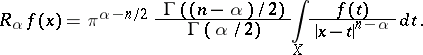The operation inverse tois said to be the Riesz derivative of order.

How to Cite This Entry:
Fractional integration and differentiation. Encyclopedia of Mathematics. URL: http://encyclopediaofmath.org/index.php?title=Fractional_integration_and_differentiation&oldid=13622
This article was adapted from an original article by P.I. Lizorkin (originator), which appeared in Encyclopedia of Mathematics - ISBN 1402006098. See original article